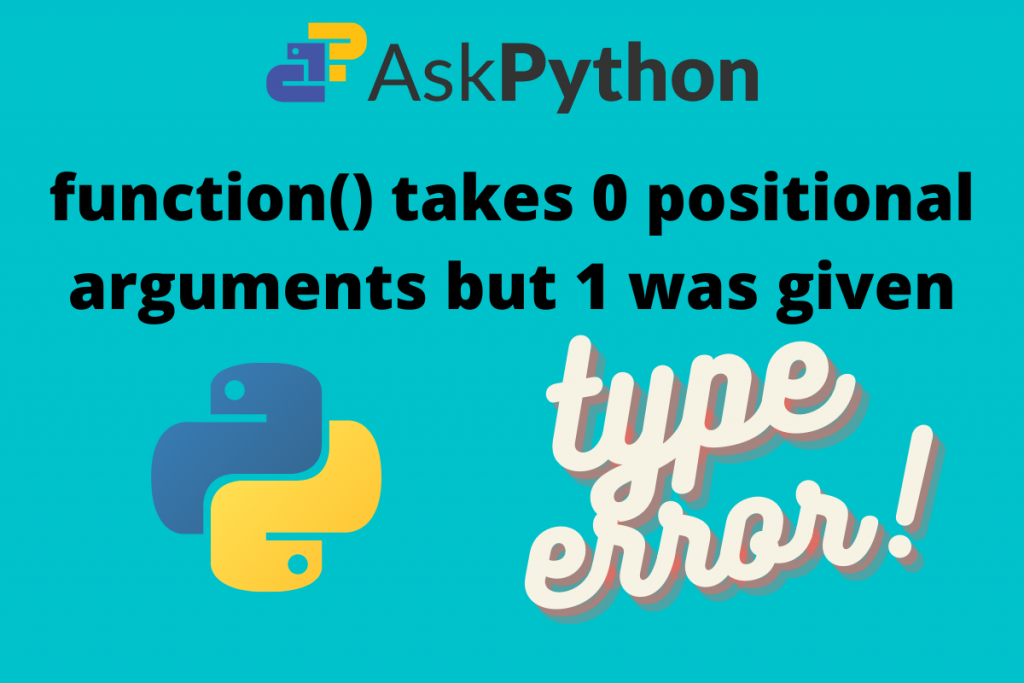# [FIXED] Takes ‘0’ positional arguments but ‘1’ was givenIn this article, we’ll learn how to fix the error “Takes 0 positional arguments but 1 was given”. Let’s get started!

## Why does “Takes ‘0’ positional arguments but ‘1’ was given” error occur?

Let’s define the following sample function “add_numbers” which accepts two arguments num_1 and num_2.

Code example 1:

```def add_numbers(num_1, num_2):
sum = num_1 + num_2
print('The sum of two numbers is: ', sum)
```

Now when we need to add two numbers, we only need to pass those numbers as arguments to the function. Take a look below:

Output:

```>>> add_numbers(33, 23) # calling the function first time
>>> The sum of two numbers is: 56
>>> add_numbers(45, 45) # calling the function second time
>>> The sum of two numbers is: 90
```

Hence, from the output, we can see that calling the function as many times is much easier than performing raw addition. Let us perform another task of multiplying two numbers.

Code example 2:

```def multiply(num_1, num_2):
product= num_1 * num_2
print('The product of two numbers is: ', product)
```

Output:

```>>> multiply(4, 100) # calling the function first time
>>> The product of two numbers is: 400
>>> multiply(40, 60) # calling the function second time
>>> The product of two numbers is: 2400
```

## Types of functions

There are two types of functions:

1. Parameterized: Values to be placed inside the parenthesis. Generally is used for the high end applications.
2. Non-parameterized: Empty parenthesis. Generally in use for basic processes.

When programmers work with parameters of a particular function they need to keep a track of some things in mind:

1. The number of parameters the function holds.
2. What each parameter is supposed to do.

When a programmer fails to consider these two points, the python interpreter raises errors. One of those is:

```Traceback (most recent call last):
File "c:\Users\Lenovo\Desktop\sample.py", line 8, in <module>
function(parameter)
TypeError: function() takes 0 positional arguments but 1 was given
```

This is the most common TypeError in Python. It occurs when the specified matching data type is not found for that particular piece of code.

### Example: Takes 0 positional arguments but 1 was given.

Let us say, we define a function to divide two numbers. It is a non-parameterized function that takes input after calling.

Example 1:

```def divide():
num_1 = int(input('enter num_1: ' )) # taking input for num_1
num_2 = int(input('enter num_2: ' )) # taking input for num_2

div = num1/num2
print('The division is: ', div)

divide(3)
```

Output:

```Traceback (most recent call last):
File "c:\Users\Lenovo\Desktop\sample.py", line 8, in <module>
divide(3)
TypeError: divide() takes 0 positional arguments but 1 was given
```

In the above case, the divide() function requires two parameters. Both the parameters are mandatory and neither of them is positional. This is why, the function throws an error “takes 0 positional arguments, but 1 was given”

When we call divide() with one parameter the interpreter throws the error.

Example 2:

```def add_numbers():
num_1 = int(input('Enter number 1: '))
num_2 = int(input('Enter number 2: '))
sum = num_1 + num_2
print('The sum of two numbers is: ', sum)

add_numbers(45, 2) #  calling the function in python shell
```

Output:

```Traceback (most recent call last):
File "c:\Users\Lenovo\Desktop\sample.py", line 7, in <module>
TypeError: add_numbers() takes 0 positional arguments but 2 were given
```

As we know that the interpreter reads the code line by line it is scanning each line of code and throws the error. We get the same error as we give two positional arguments despite the function accepting nothing.

## How to Fix “Takes ‘0’ positional arguments but ‘1’ was given” Error?

The error will display the function name where the error occurs. To fix the error:

• Check what type of parameters the functions accepts
• Find all the calls for that function and identify if any of the function call is incorrectly made
• Fix the error by simply changing the function call in question

## Conclusion

The topic of “takes 0 positional arguments but 1 was given” ends here. The concept is straightforward. I hope you were able to fix the error in your code. IF you have any questions, drop us a line and we’ll help you out.